MATHEMATICS

EXAM BOARD

EDEXCEL

STRUCTURE OF COURSE

Pure Mathematics 1

Pure Mathematics 2
Statistics and Mechanics

ASSESSMENT

Each component is assessed by a written examination lasting 2 hours and all units carry equal weighting.

Each paper is 100 marks and all exams will be sat in the summer of 2022 Year 13

CONTENT

Component 1

Pure Mathematics 1

Proof, Algebra and functions, Coordinate geometry in the (x,y) plane, Sequences and series, Trigonometry, Exponentials and logarithms, Differentiation, Integration, Vectors.

Component 2

Pure Mathematics 2

Proof, Algebra and functions, Coordinate geometry in the (x,y) plane, Sequences and series, Trigonometry, Differentiation, Integration, Numerical methods

Component 3

Statistics and Mechanics

Section A: Statistics: Statistical sampling, Data presentation and interpretation, Probability, Statistical distributions, Statistical hypothesis testing

Section B: Mechanics: Quantities and units in mechanics, Kinematics, Forces and Newton’s laws, Moments.

POSSIBLE CAREER PATHS

Mathematics at Advanced Level is a very sought after qualification and is in fact an essential requirement for many university courses. It demonstrates that you have the ability to think logically, are numerate and can solve problems analytically. Possible careers include those in Engineering, Medicine, Actuarial Science, Financial and IT related professions.

POSSIBLE SUBJECT COMBINATIONS

 Mathematics is itself a highly regarded subject but also supports the learning in several other subjects, notably the Sciences, Economics, Psychology and Geography. Some universities prefer candidates to have studied Further Mathematics so you should consider this carefully as an option if you are thinking of studying Mathematics, Engineering or Economics at tertiary level.
•••••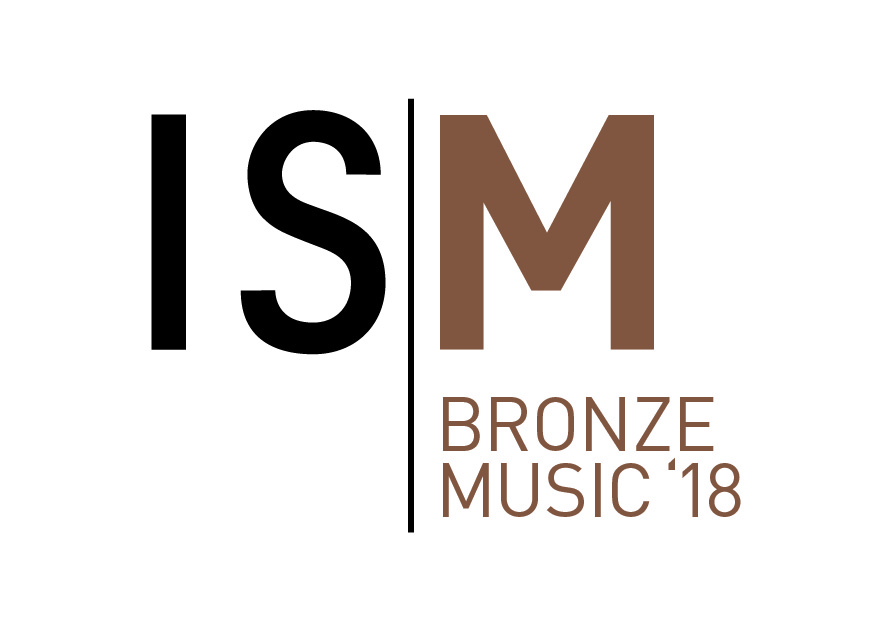••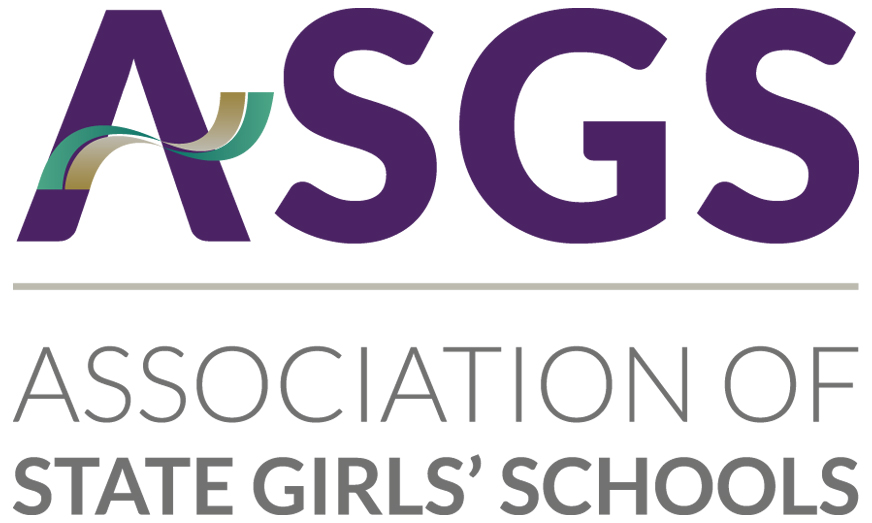•••••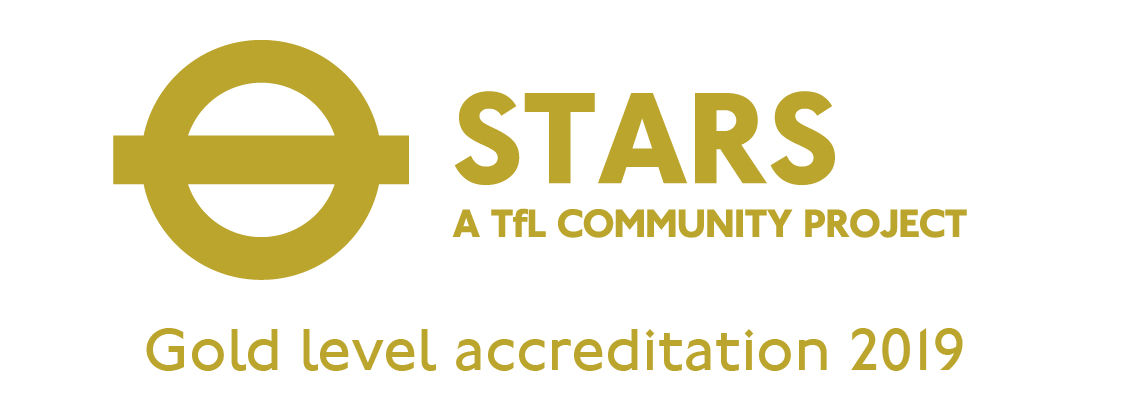•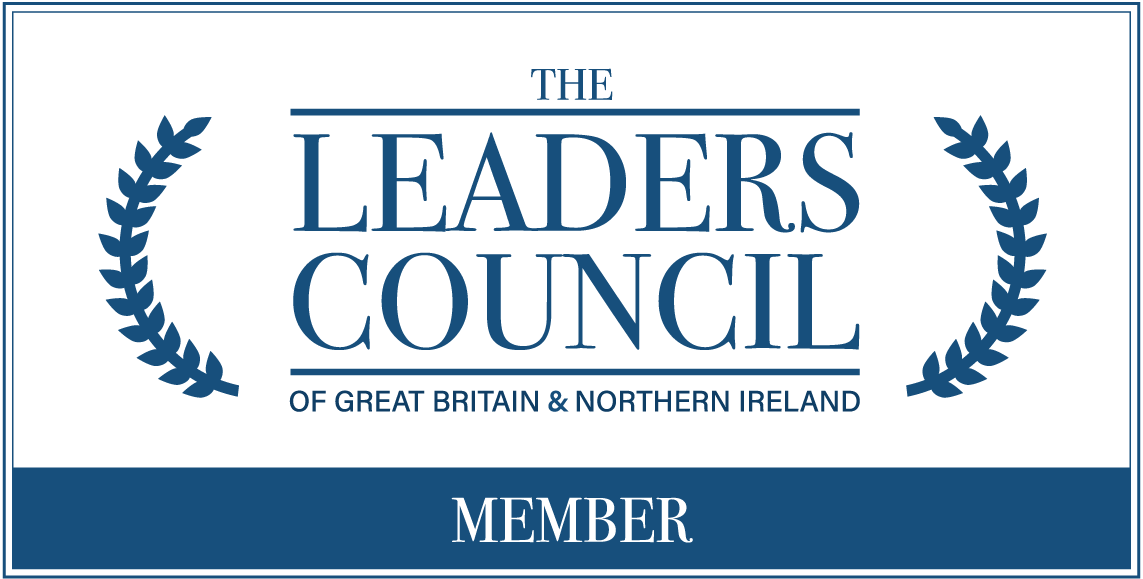••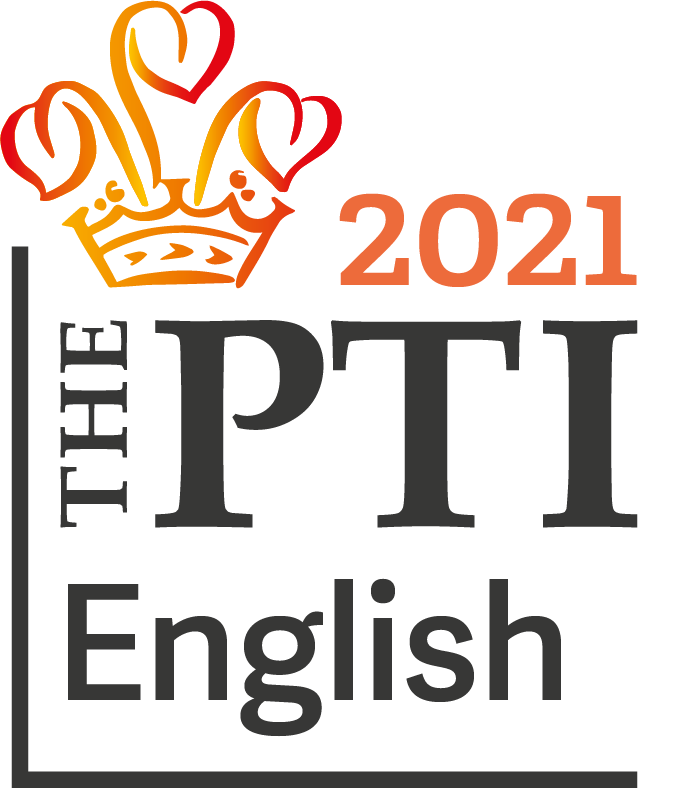•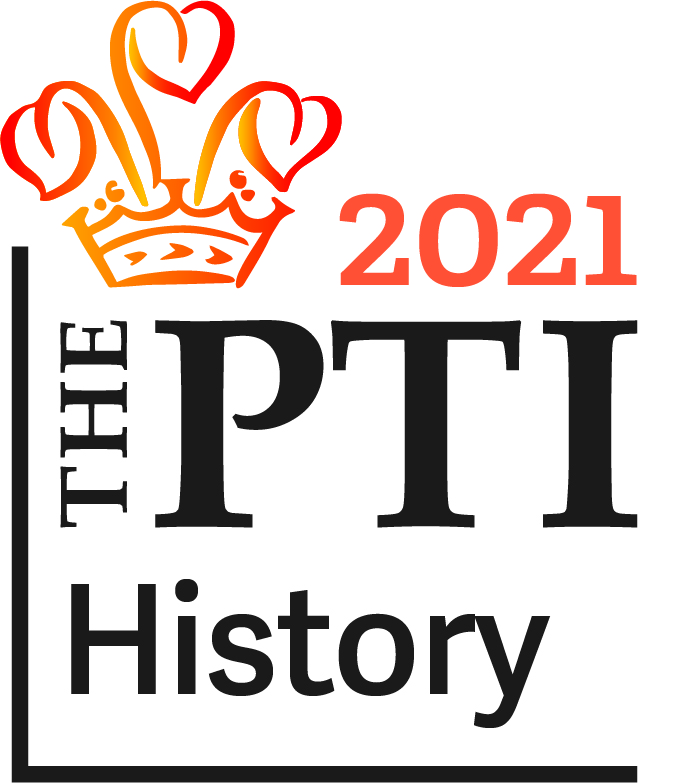•